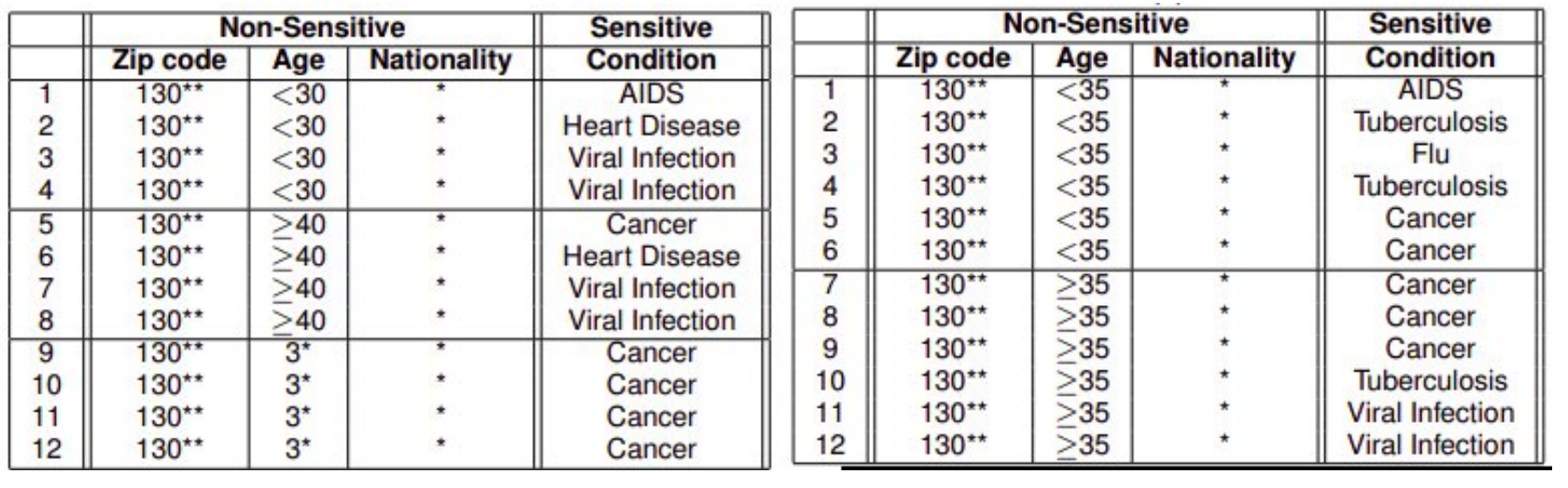# L1-Some Attempts at Data Privacy

## 纽约市出租车数据集泄露问题

### 案发经过：

• 纽约市向市民公开了一份出租车大数据集
• 其中所有数据都没有标出具体的车牌号码，而采用一个唯一标识码（medallion number）去掩盖
• 但是其中发现了一个人的收入远远高于其他出租车司机
• 经过查表$$MD5$$发现，这个人的唯一标识码（cfcd208495d565ef66e7dff9f98764da）就是$$0$$，也就是缺失值
• 因为司机的车牌号码都是短短的几个字母，为此可以通过快速的查表$$MD5$$查出结果

### 问题反思：

• 有人提出不用原数据生成这项唯一标识码，而是重新生成一组随机的数字作为唯一标识码，是否可行？
• 显然是依旧存在问题的，假若你乘搭某位司机的出租车之后，记录下具体的位置以及时间及其对应的车牌号码。再重新对发布的数据集进行关联分析，极易就能找出这位司机的敏感隐私信息

## 网飞奖金赛

### 案发经过：

• 网飞举办一个奖金赛：提供训练集以向参赛者募集最为强大的推荐模型
• 网飞官方的提供的数据集都进行了匿名化处理，这是依据法律必须做的
• 但是这样的作法并非万无一失，攻击者通过用IMDb得到评论数据集（没有匿名化的），再对网飞发布的匿名数据集进行关联分析
• 即得到了网飞数据集中每一个数据的作成者

### 问题反思：

• 显然通过匿名化处理的数据集弱不禁风，完全不足以保护隐私

## 神经网络的记忆问题

### 攻击手段

• 针对一个基于词袋$$Y$$训练好，特定的自然语言模型$$f_\theta$$，给定一个特定的序列$$x_1,\dots,x_n$$

• 那么给出$$log-perplexity$$的定义

$P_\theta(x_q, \dots,x_n)=-\log_2Pr(x_1,\dots,x_n | f_\theta)=\sum^n_{i=1}(-\log_2Pr(x_i|f_\theta(x_1,\dots,x_{i-1})))$

• 这个指数在对于敏感数据时会呈现出低的分数

## $$k$$-匿名化（$$k$$-anonymity）posted @ 2021-03-07 21:02  Uzuki  阅读(357)  评论(0编辑  收藏  举报